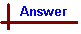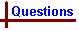CHEMICAL EQUATIONS (Questions) Shortcut to Questions Q: 1 2 3 4 1 Write ionic equations for any reaction that occurs when the following pairs of substances are mixed. If no reaction occurs, write "no reaction". (1.) a water solution of sodium sulfate and a water solution of barium chloride (2.) a water solution of potassium hydroxide and a water solution of copper(II) nitrate (3.) a water solution of silver nitrate and a water solution of sodium chloride (4.) a water solution of silver nitrate and a water solution of barium chloride (5.) a water solution of silver nitrate and dilute hydrochloric acid (6.) a water solution of lead(II) nitrate and a water solution of potassium chloride (7.) a water solution of copper(II) chloride and a water solution of lead(II) nitrate (8.) a water solution of iron(III) sulfate and a water solution of sodium hydroxide (9.) a water solution of cobalt(II) sulfate and a water solution of ammonium carbonate (10.) a water solution of sodium phosphate and a water solution of magnesium chloride (11.) a water solution of magnesium bromide and a water solution of lead(II) nitrate (12.) a water solution of copper(II) nitrate and a water solution iron(III) sulfate (13.) a water solution of potassium iodide and a water solution of sodium chloride (14.) a water solution of aluminium sulfate and a water solution of potassium phosphate2 Write an ionic equation for any reaction that occurs when the following pairs are mixed. If no reaction occurs, write "no reaction". (1.) sodium carbonate and dilute nitric acid (2.) a water solution of sodium carbonate and dilute nitric acid (3.) copper(II) carbonate and dilute sulfuric acid (4.) copper(II) carbonate and dilute hydrochloric acid (5.) silver carbonate and dilute nitric acid (6.) iron(II) hydroxide and dilute hydriodic acid (7.) sodium hydroxide and dilute nitric acid (8.) a water solution of sodium hydroxide and dilute sulfuric acid (9.) sodium hydroxide and dilute hydrochloric acid (10.) magnesium oxide and dilute hydrochloric acid (11.) sodium hydroxide and dilute hydrobromic acid (12.) a water solution of barium hydroxide and a water solution of ammonium chloride (13.) a water solution of ammonium sulfate and a water solution of sodium hydroxide (14.) a water solution of calcium hydrogencarbonate and dilute solution of hydrochloric acid (15.) a water solution of sodium sulfite and excess dilute hydrochloric acid3 Write an equation for the reaction that occurs when an excess of warm 4 M nitric acid is added to each of the following. If no reaction occurs, write "no reaction". (1.) barium sulfate (2.) ammonium nickel(II) sulfate-6-water (3.) aluminium hydroxide (4.) calcium carbonate4 How would you produce water solutions of the metal ions of the following substances? Briefly describe the method to be used and write ionic equations for the reactions involved. (1.) barium carbonate (2.) iron(III) hydroxide (3.) zinc (4.) sodium (5.) manganese(II) sulfide (6.) copper(II) oxide (7.) strontium sulfiteCHEMICAL EQUATIONS (Answers) 1 The question specifies ionic equations to be written, so only the species directly involved in the reaction are noted (ie spectator ions are left out, since they appear on both sides of the equation and do not contribute to any reaction). Any species in water solution is "aquated" - i.e. it is associated with a number of water molecules which are held to the dissolved molecule, atom or ion by intermolecular attractive forces. Often this is also shown in ionic equations by writing such species with an (aq) attached, for example, Mg2+(aq) for a magnesium ion. However, this practice is not generally observed here because it is understood that all species in a water solution are in fact aquated. No reaction occurs if the ions present after mixing the two solutions are exactly the same as those present in the two solutions before mixing without formation of a precipitate, gas, complex or any new species. Equations for reactions are given below: As usual, it is customary to balance the equations whether specified in the question or not. (1.) Ba2+ + SO42- → BaSO4(s) (2.) Cu2+ + 2OH- → Cu(OH)2(s) (3.) Ag+ + Cl- → AgCl(s) (4.) Ag+ + Cl- → AgCl(s) (5.) Ag+ + Cl- → AgCl(s) (6.) Pb2+ + 2Cl- → PbCl2(s) (7.) Pb2+ + 2Cl- → PbCl2(s) (8.) Fe3+ + 3OH- → Fe(OH)3(s) (9.) Co2+ + CO32- → CoCO3(s) (10.) 3Mg2+ + 2PO43- → Mg3(PO4)2(s) (11.) Pb2+ + 2Br- → PbBr2(s) (12.) No reaction (13.) No reaction (14.) Al3+ + PO43- → AlPO4(s)2 (1.) Na2CO3(s) + 2H+ → 2Na+ + H2O + CO2(g) (2.) (a) CO32- + H+ → HCO3-, (b) CO32- + 2H+ → H2O + CO2(g) (3.) CuCO3(s) + 2H+ → Cu2+ + H2O + CO2(g) (4.) CuCO3(s) + 2H+ → Cu2+ + H2O + CO2(g) (5.) Ag2CO3(s) + 2H+ → 2Ag+ + H2O + CO2(g) (6.) Fe(OH)2(s) + 2H+ → Fe2+ + 2H2O (7.) NaOH(s) + H+ → Na+ + H2O (8.) OH- + H+ → H2O (9.) NaOH(s) + H+ → Na+ + H2O (10.) MgO(s) + 2H+ → Mg2+ + H2O (11.) NaOH(s) + H+ → Na+ + H2O (12.) NH4+ + OH- → NH3(g) + H2O (13.) NH4+ + OH- → NH3(g) + H2O (14.) HCO3- + H+ → H2O + CO2(g) (15.) SO32- + 2H+ → H2O + SO2(g)3 (1.) No reaction. Barium sulfate is very insoluble, so the equilibrium favours the reactants over the formation of any products. (2.) No reaction with the acid but solid dissolves in the water present in the acid solution: (NH4)2Ni(SO4)2.6H2O(s) → 2NH4+ + Ni2+ + 2SO42- + 6H2O (3.) Al(OH)3(s) + 3H+ → Al3+ + 3H2O (4.) CaCO3(s) + 2H+ → Ca2+ + CO2(g) + H2O4 (1.) Add excess dilute hydrochloric acid or excess dilute nitric acid (boil off carbon dioxide formed): BaCO3(s) + 2H+ → Ba2+ + H2O + CO2(g). Solubility considerations are important here - a number of other acids would be suitable for this reaction, but not sulfuric acid since the insoluble compound, barium sulfate, would form, leaving no Ba2+ in solution! (2.) Add excess dilute acid: Fe(OH)3(s) + 3H+ → Fe3+ + 3H2O (3.) Add excess dilute acid: Zn(s) + 2H+ → Zn2+ + H2(g) (4.) Add to excess water (dangerous!): 2Na(s) + 2H2O(l) → 2Na+ + 2OH- + H2(g) or Add to excess ethanol (safer): 2Na(s) + 2C2H5OH(l) → 2Na+ + 2C2H5O- + H2(g). The dissolved sodium ethoxide hence formed can then be added to water to provide a water solution of sodium ions without the hazard of reacting sodium with water directly. (5.) Add excess dilute acid (boil off hydrogen sulfide formed): MnS(s) + 2H+ → Mn2+ + H2S(g) (6.) Add excess dilute acid (heat to promote reaction): CuO(s) + 2H+ → Cu2+ + H2O (7.) Add excess dilute hydrochloric acid or dilute nitric acid (boil off sulfur dioxide formed). SrSO3(s) + 2H+ → Sr2+ + H2O + SO2(g)